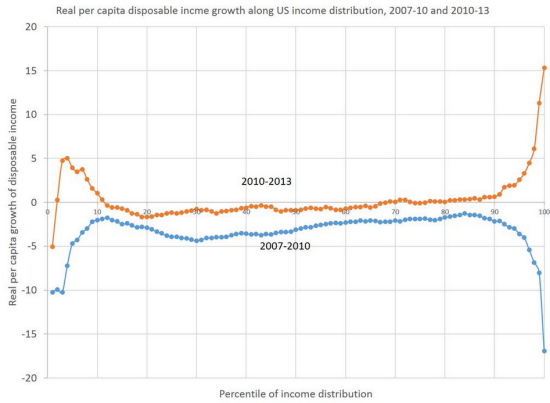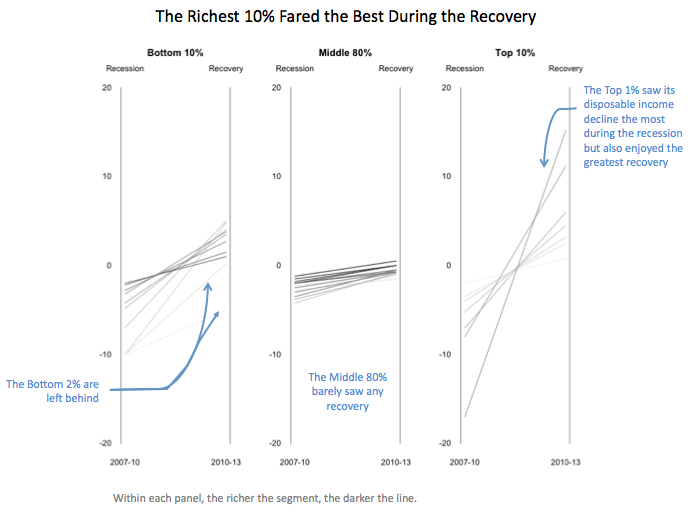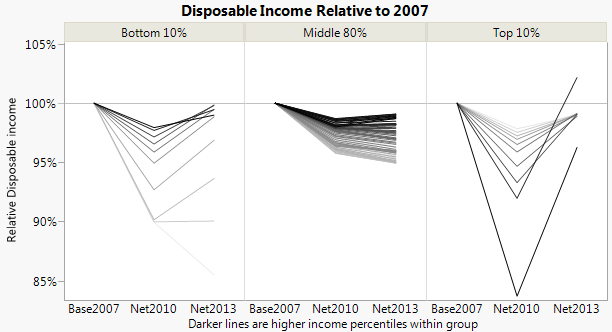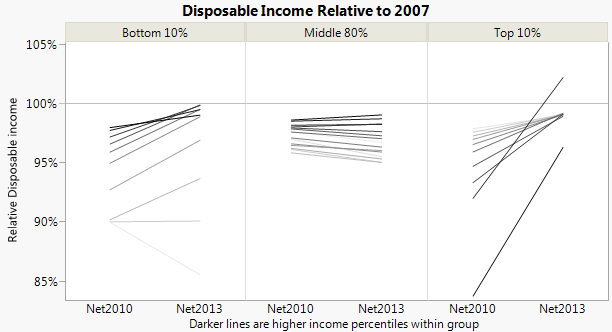Our World Statistics Day conversations have been a great reminder of how much statistics can inform our lives. Do you have an example of how statistics has made a difference in your life? Share your story with the Community!
Choose Language Hide Translation Bar
Graph makeover: Disposable income change chart

Kaiser Fung recently critiqued this chart of changes on disposable income.He put forth the idea of using categorized slope graphs instead:How do we do something like that in JMP? We can do paneling and lines with variable color in Graph Builder; we just need to get the data into the right form. As usual, a key step in making a slope graph in Graph Builder is using Table > Stack to get the two data columns into a column of labels and a column of data. Then the label column goes on the X axis, and the data column goes on the Y axis.

First, we need to get the data. Sometimes when I'm doing a quick remake, the simplest thing to do is the read the data right off the graph. There are programs and websites to help with that. This time, I used the online app, Web Plot Digitizer, which produced a separate CSV file for each series. The values are only estimates of the original values, of course.

After opening the two CSV files and setting the column names to "x" and "y" I wrote the following script to combine them and calculate some derived values.

``````dt1 = Data Table( "data" );

dt2 = Data Table( "data (1)" );

dt = New Table( "changes",
New Column( "Percentile", Format( "Percent", 8, 0 ), Set Values( (1 :: 100) / 100 ) ),
New Column( "Base", Format( "Percent", 8, 1 ) ),
New Column( "Change2010", Format( "Percent", 8, 1 ) ),
New Column( "Change2013", Format( "Percent", 8, 1 ) ),
New Column( "Net2010", Format( "Percent", 8, 1 ) ),
New Column( "Net2013", Format( "Percent", 8, 1 ) ),
New Column( "Group", Character ),
New Column( "Rank" )
);

For Each Row(
dt,
dt:Base = 1.0;
dt:Change2010 = Interpolate( dt:Percentile, dt2:x << Get Values(), dt2:y << Get Values );
dt:Change2013 = Interpolate( dt:Percentile, dt1:x << Get Values(), dt1:y << Get Values );
dt:Net2010 = dt:Base * (1 + dt:Change2010);
dt:Net2013 = dt:Net2010 * (1 + dt:Change2013);
dt:Group = If(
dt:Percentile <= 0.1, "Bottom 10%",
dt:Percentile <= 0.9, "Middle 80%",
"Top 10%"
);
dt:Rank = If(
dt:Percentile <= 0.1, dt:Percentile / .1,
dt:Percentile <= 0.9, (dt:Percentile - .1) / .8,
(dt:Percentile - .9) / .1
);
);``````

The interpolation is to align the "x" values on even percentile values. The Group and Rank are to control how we group and color in Graph Builder. The Base and Net values are because I wanted to try something a little different than what Kaiser did. Just seeing loss and gain percentages is not enough to see the net effect, so I explicitly compute the net effect and graph those instead.

Note: This calculation comes with a big if: It assumes the percentiles are the same across both periods. That is, that the people in a given percentile for the 2007-2010 period are in the same percentile for the 2010-2013 period. I don't know if that's the case, though it's unlikely the percentiles changed too much in the first period given every percentile went down and at mostly uniform rates.

After stacking the three columns, Base2007, Net2010, and Net2013, I can create the lines chart. I put Group into the Group X role and Rank into the Color role, added a reference line at 100% and updated the labels.I just realized that even though I need the base value for the calculations, I don't actually need to show the base values (they're all the same). After using a Local Data Filter to exclude Base2007 and following Kaiser's example to thin out the middle group, I get:Article Labels

There are no labels assigned to this post.

Article Tags
1 Comment
Visitor

Charlie Shipp wrote:

This is great work. Waking up this morning, I thought of

something similar (a time-plot) for our personal finance.